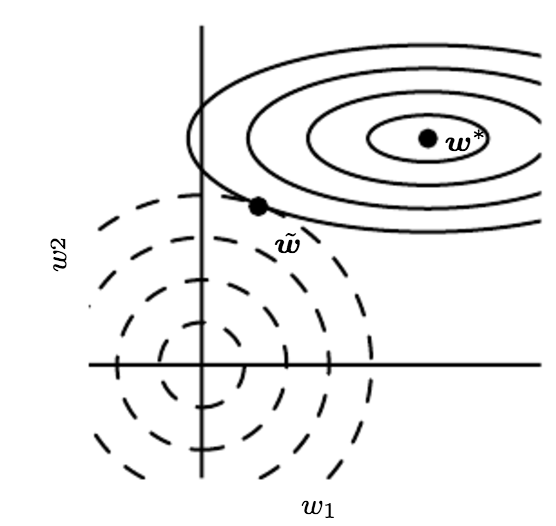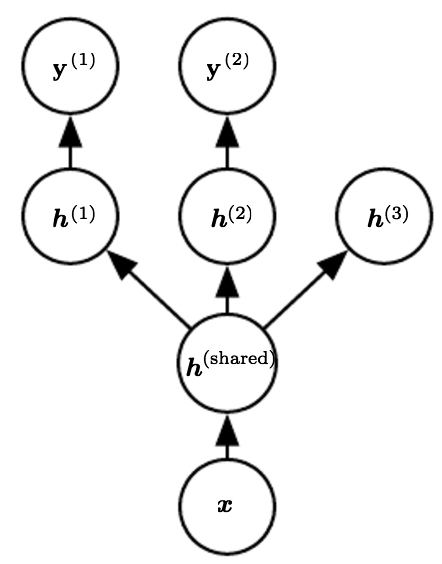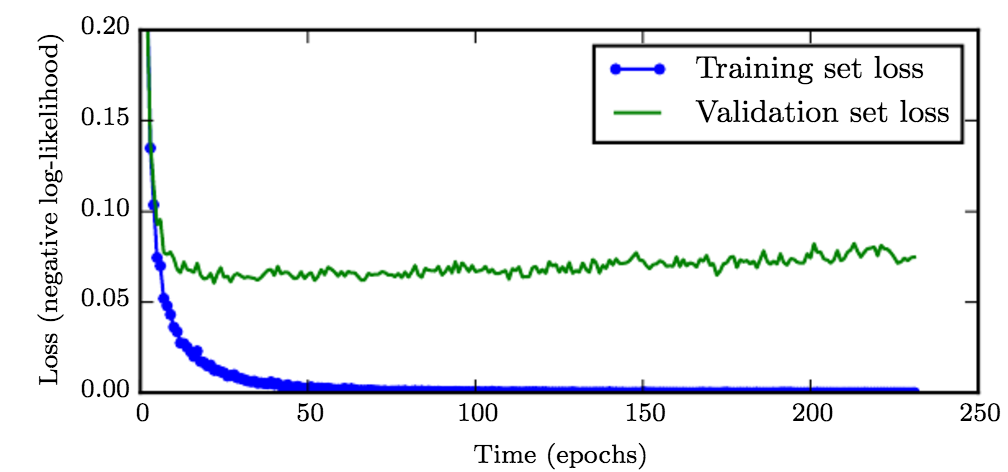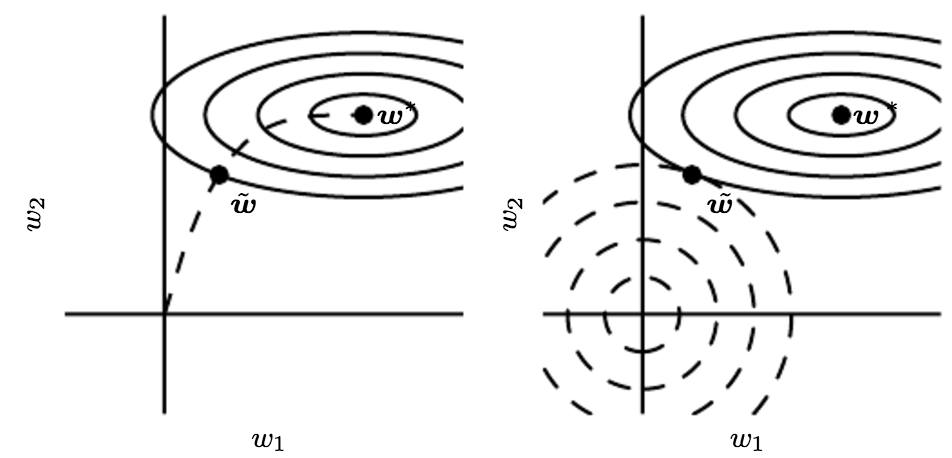# 参数范数惩罚

## L2参数正则化

L2参数正则化之后的模型具有以下总的目标函数：

1. 当$\lambda_i \gg \alpha$时，惩罚因子作用比较小。
2. 当$\lambda_i \ll \alpha$时，对应的参数会缩减至0。## L1参数正则化

L2权重衰减是权重衰减最常见的形式，我们还可以使用其他的方法限制模型参数的规模。 比如我们还可以使用L1参数正则化。

## L1正则化和L2正则化的区别

1. 通过上面的分析，L1相对于L2能够产生更加稀疏的模型，即当L1正则在参数w比较小的情况下，能够直接缩减至0.因此可以起到特征选择的作用，该技术也称之为 LASSO
2. 如果从概率角度进行分析，很多范数约束相当于对参数添加先验分布，其中L2范数相当于参数服从高斯先验分布；L1范数相当于拉普拉斯分布。
L2正则化 L1正则化# 半监督学习

{Russ+Geoff-nips-2007}描述了一种学习回归核机器中核函数的方法，其中建模$P(x)$时使用的未标记样本大大提高了$P(y \mid x)$的效果。

# 多任务学习# 提前终止Let $n$ be the number of steps between evaluations.
Let $p$ be the “patience,” the number of times to observe worsening validation set error before giving up.
Let $\theta_0$ be the initial parameters.
$\theta \leftarrow \theta_0$
$i \leftarrow 0$
$j \leftarrow 0$
$v \leftarrow \infty$
$\theta^{\ast} \leftarrow \theta$
$i^{\ast} \leftarrow i$
while $j < p$ do
Update $θ$ by running the training algorithm for $n$ steps.
$i ← i + n$
$v’← ValidationSetError(θ)$
if $v’< v$ then
$j ← 0$
$θ^{\ast}← θ$
$i^{\ast}← i$
$v ← v’$
else
$j ← j + 1$
end if
end while
Best parameters are $θ^{\ast}$, best number of training steps is $i^{\ast}$

• 提前停止策略使用起来非常方便，不需要改变原有损失函数，简单而且执行效率高。但是它需要一个额外的空间来备份多份模型参数。
• 提前停止策略可以和其他正则化策略一起使用。
• 提前停止策略确定训练迭代次数后，有两种策略来充分利用训练数据，一是将全量训练数据一起训练一定迭代次数；二是迭代训练流程直到训练误差小于提前停止策略的验证误差。
• 对于二次优化目标和线性模型，提前停止策略相当于L2正则化。# 稀疏表示

L1惩罚可以诱导稀疏的参数，即许多参数为零（或接近于零）。

# 集成化方法（Ensemble Methods）

Bagging是一种常用的集成学习方法。Bagging的策略很多，例如不同初始化方法、不同mini batch选择方法、不同的超参数选择方法。

# Dropout

Dropout方法提供了正则化一大类模型的方法，计算方便但功能强大。其基本思路非常简单：在训练阶段，对于每个mini-batch随机抹去一定比例的神经元进行前向及后向传播更新, 如下图所示。1. 随机选择10-20个屏蔽向量就可以得到一个较好的解。
2. 采用几何平均然后在归一化的思路，从而达到平滑的效果。

Dropout还可以看作是一种在隐藏层注入掩码噪声的方法。了解这一事实是重要的, 这可以看作是对输入内容的信息高度智能化、自适应破坏的一种形式，而不是对输入原始值的破坏。